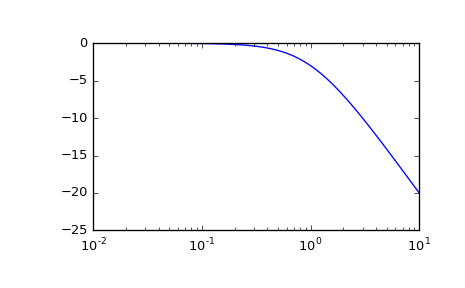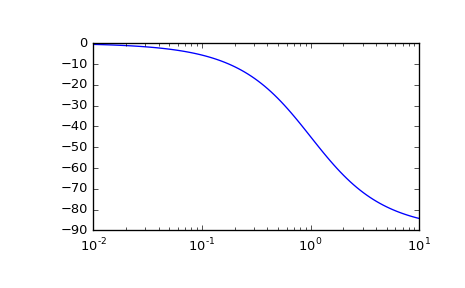# scipy.signal.bode¶

scipy.signal.bode(system, w=None, n=100)[source]

Calculate Bode magnitude and phase data of a continuous-time system.

Parameters: system : an instance of the LTI class or a tuple describing the system. The following gives the number of elements in the tuple and the interpretation: 1 (instance of lti) 2 (num, den) 3 (zeros, poles, gain) 4 (A, B, C, D) w : array_like, optional Array of frequencies (in rad/s). Magnitude and phase data is calculated for every value in this array. If not given a reasonable set will be calculated. n : int, optional Number of frequency points to compute if w is not given. The n frequencies are logarithmically spaced in an interval chosen to include the influence of the poles and zeros of the system. w : 1D ndarray Frequency array [rad/s] mag : 1D ndarray Magnitude array [dB] phase : 1D ndarray Phase array [deg]

Notes

If (num, den) is passed in for system, coefficients for both the numerator and denominator should be specified in descending exponent order (e.g. s^2 + 3s + 5 would be represented as [1, 3, 5]).

New in version 0.11.0.

Examples

>>> from scipy import signal
>>> import matplotlib.pyplot as plt

>>> sys = signal.TransferFunction(, [1, 1])
>>> w, mag, phase = signal.bode(sys)

>>> plt.figure()
>>> plt.semilogx(w, mag)    # Bode magnitude plot
>>> plt.figure()
>>> plt.semilogx(w, phase)  # Bode phase plot
>>> plt.show()#### Previous topic

scipy.signal.freqresp

#### Next topic

scipy.signal.dlti